# Estimation of the term premium within Australian Treasury Bonds

The following is a working paper prepared as part of the AOFM’s research program which explains the methodology behind the Term Premium Estimates published in the Data Hub. Readers should be aware that the strategies and conclusions presented may no longer reflect the current debt management practices used by the AOFM.

AOFM Working Paper 2017-01 (PDF)

## Estimation of the term premium within Australian Treasury Bonds

Australian Office of Financial Management Working Paper 2017-01

Fraser Jennison

Portfolio Strategy and Research, The Australian Office of Financial Management

Treasury Building, Langton Crescent Parkes ACT 2600

## Abstract

We explore a linear-regression based dynamic term structure model developed by Adrian, Crump and Moench ‘ACM’ (2013). We fit this model to the Australian Treasury Bond term structure and estimate term premium through a daily yield decomposition. While our estimation approach follows ACM, we consider an alternative estimation approach that corrects for the potential bias inherent within a vector autoregressive system when variables are highly persistent. Our findings show that this alternative specification leads to meaningfully different term premium estimations and more variability in risk-neutral yields, while both estimation techniques suggest that the secular decline in yields experienced within Australia has primarily been driven by a falling term premium. To address estimation uncertainty, we provide yield decompositions with and without this alternative estimation approach and suggest that the true data generating process most likely lies somewhere within the bounds of these model specifications.

## 1. Introduction

Once found primarily within academic literature, the term premium has become a more widely discussed concept. Representing the excess return investors demand for holding a longer-term bond as opposed to investing in a series of short term bonds, this concept has materialised in many settings including sovereign debt management, economic forecasting, and within central bank policy discussions, particularly in relation to quantitative easing programs. To measure the term premium, government bond yields need to be decomposed into two components: (1) a component based on the expected future path of short term rates; and (2) a term premium component. As market expectations are not directly observable, econometric techniques are required to separate the term premium from expectations.

For robust term premium estimation, an econometric model that estimates a time-varying term premium based on multiple dimensions of the yield curve is required. Campbell and Shiller (1988) found evidence that rejects a time-invariant term premium, making static risk premia estimation approaches such as the Fama MacBeth (1973) regression technique unfeasible in this context. In the early bodies of literature, such as Vasicek (1977) or Cox, Ingersoll and Ross (1985), the evolution of interest rates is explained by movements in the instantaneous spot rate. While specifying a single factor model has the advantage of simplicity, information encapsulated within the entirety of the yield curve is absent. More recent models reduce the yield curve into multiple factors, whether these are latent variables extracted from the Nelson Siegel (1987) functional form or through principal component analysis. While multi-factor models can accurately capture yield dynamics, they may require computationally challenging non-linear cross-sectional restrictions which may in themselves be problematic. Adrian, Crump and Moench ‘ACM’ (2013) propose a multi-factor affine dynamic term structure model (DTSM) with coefficient estimation through a series of linear-regressions. This approach can estimate a time-varying term premium, based on multiple factors, without imposing non-linear restrictions using a three-step linear regression approach.

Preceding ACM, seminal literature such as Duffie and Kan (1996), Dai and Singleton (2002), Duffee (2002) and Kim and Wright (2005) have made contributions to the development of multifactor-affine DTSM models. Within an Australian context: Finlay and Chambers (2009) fit a term structure model to the Australian yield curve, Finlay and Wende (2011) fit a term structure model to Australian Indexed bond yields and Wright (2011) includes Australia in an international term premia empirical study. While DTSMs allow for decomposition of yields into a term premium and a risk-neutral yield component that represents expectations for inflation and real yields, estimation difficulties can arise. For example, it is known that parameter estimation within the vector autoregressive system that represents interest rate dynamics can be difficult to identify. This identification problem can cause a decomposition of yields to suffer from a bias if estimated through a technique such as ordinary least squares (Bauer et al., ‘BRW’, 2012). This potential bias is a function of the persistent nature of interest rates (slow-mean reversion) and the sample size of data available (number of interest rate cycles captured). To account for this, BRW suggest that the ordinary least squares VAR parameters within a DTSM should be bias-corrected which usually results in estimated risk-neutral rates exhibiting more variability (greater persistency). Overall, making a bias-correction within a DTSM typically leads to more variation in estimates of risk-neutral yields and consequently more stable estimates of term premia.

This paper decomposes the Australian Treasury Bond yield curve in order to estimate the term premium using the linear-regression based approach developed by Adrian, Crump and Moench (2013). While our estimation approach follows ACM, we recognise the uncertainty around estimation of the vector autoregressive system that ultimately represents interest rate dynamics in a DTSM. As such, we provide two yield decompositions: (1) leaving the OLS VAR parameters unadjusted as per ACM; and (2) using a bias-correcting technique to adjust the VAR parameters for potential bias. We suggest that given estimation uncertainty, the true data-generating process most likely lies somewhere within these model specifications. While this paper does not suggest an optimal estimation approach, we do suggest that development of an optimisation procedure in order to find a preferred combination of these alternative approaches could be valuable in managing the uncertainty of DTSM coefficient estimations and consequently, term premium estimation.

In Section 2 we provide an overview of the ACM model, introduce the regressions used for estimation and the data used for decomposing the Australian Treasury Bond term structure. Section 3 discusses a potential issue of DTSM parameter estimation as a consequence of the persistency of interest rates, proposing a technique to address this concern. Section 4 analyses the optimal number of factors to include in this model. Section 5 explores model results and Section 6 concludes.

## 2. The Model

The method we use to decompose the Australian Treasury Bond term structure is the linear regressions approach presented by Adrian, Crump and Moench (2013). An overview of the model is presented in this section; for more detail see ACM.

### 2.1 Expression

ACM derive an expression for continuously compounded arbitrage free excess holding period returns from a dynamic term structure model with an affine pricing kernel. Within this expression, the dynamics of interest rates are expressed as state variables that evolve following an autoregressive process that contains both random diffusion terms and non-random mean reverting drift terms, that is:The value of  (representing the shocks to the state variables) follows a Gaussian distribution with variance-covariance matrix :The model also assumes that there exists an exponentially affine pricing kernel Mt which discounts the expected future price of a zero-coupon bond paying one unit at time t + n (Pt+1(n-1)) to the price in the current period, that is:The pricing kernel is an exponential function of the risk free rate, the market price of risk and the shocks to the state variables, which links the risk-neutral and real-world probability distributions. It can be expressed as:Following Duffee (2002), the market price of risk λt is assumed to be an affine function of the state variables, that is:Combining these elements, excess holding period returns can be expressed as a function of the bonds’ expected return, a convexity adjustment, the (priced) shocks to the state variables and an orthogonal return pricing error, that is:Written for a portfolio of bonds across different maturities and time periods: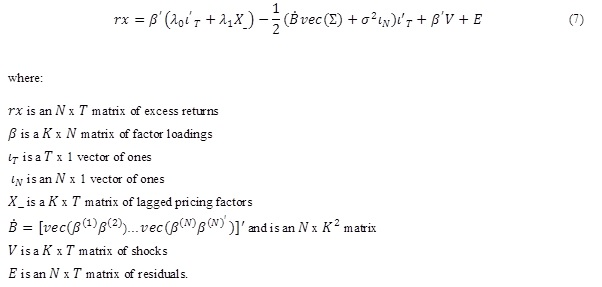### 2.2 Estimation

The model parameters can be estimated through linear regressions as detailed in ACM and outlined below. We take the same approach to parameter estimation with exception to the vector autoregression (VAR) process. We consider an alternative estimation approach which addresses a potential bias inherent within a vector autoregressive system when variables are highly persistent. This alternative estimation technique is described in section three.

### 2.2.1 Vector Autoregression

The state variables which represent a multidimensional, reduced form evolution of the yield curve are expressed by a set of K (demeaned and normalised) principal components extracted from a panel of zero-coupon Australian Treasury Bond data.

The state variables Xt+1 (a K x N matrix of principal components) are decomposed into a predictable component and an estimated shock component by the following first order vector autoregression:### 2.2.2 Time series regression

Excess returns are regressed on a constant, the lagged state variable and the estimated shock components collected from the vector autoregression.This provides estimates for the amount by which returns change in response to a constant, the lagged state variable and the shock components in the current period. The regressors are arranged in matrix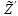such that:The least squares estimates can be written as:A variance-covariance matrix () is constructed from the residuals and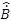is constructed from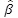as detailed in Section 2.1.

### 2.2.3 Cross sectional regression

The market price of risk is expressed in two separate components, a component that is conditionally correlated to the shock component denotedand a component that is conditionally orthogonal to the shock component denoted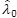. The former component can be thought of as the time-varying pricing component of market risk whereas the latter represents the time invariant component of market risk. In the third step, cross-sectional regressions provide an estimate of both market price of risk components and are obtained by the ACM expression introduced in 2.1:The estimator of the time variant market price of risk component:The estimator of the time variant market price of risk component:### 2.2.4 Recursive function

From these estimated parameters, a zero-coupon yield can be generated. We begin by expressing bond prices as an exponentially affine function of the state variables:Since the excess return for a bond purchased with n periods to maturity and sold with n - 1 periods to maturity is:excess returns can be written as:This expression can be re-written as the sum of the bond’s expected return, a convexity adjustment, the (priced) shocks to the state variables and an orthogonal return pricing error (as per the final equation in the estimation section), that is:Estimated parameters from the three step regression are the recursive function inputs that are used to calculate model-fitted bond yields. The equations are recalculated with λ0 and λ1 (the market prices of risk) set to zero, to generate the risk-neutral yield curve. The term premium is the difference between the model estimated yields and risk-neutral yields.

### 2.3 Data

We use the Reserve Bank of Australia published zero-coupon interest rates analytical series, from mid-1992 to end-2016. Construction of this series is based on the Merrill Lynch Exponential Spline methodology, for further details see Finlay and Olivan (2012). As this analytical series presents zero-coupon yields in quarterly tenor intervals, we use the Svensson (1994) extended version of Nelson and Siegel (1987) functional form to cast interest rates into tenors with monthly intervals.

## 3. Interest Rate Persistency and the Autoregressive Process

While the ACM approach allows for decomposition of yields into an expectations component (risk-neutral yields) and a term premium, parameter estimation can come with uncertainty. In particular, DTSM literature recognises the potential for biased coefficients when estimating parameters within a highly persistent vector autoregressive system, such as a system that represents the dynamics of interest rates. The potential for bias is a function of the persistent nature of interest rates (slow-mean reversion) and the sample size of data available (number of interest rate cycles captured) which make OLS estimation difficult. For a DTSM, should a small sample bias be captured within the estimated VAR parameters, interest rates will be estimated to evolve with less persistency in comparison to the true behaviour of interest rates. This has implications for risk-neutral rates which will be estimated to be spuriously stable as a consequence of faster mean reversions and less deviation from mean levels. Moreover, this has consequences for the other component of the yield decomposition: term premium estimation. Term premia will be estimated with greater variability than that which would occur under the true data generating process. While this issue has been identified in literature, attempts to correct for biases within term structure models and uncover the implication for term premium estimation are less common. This largely is a reflection that many bias-correction techniques are based on simulations which were impractical before linear regression-based term structure modelling approaches, such as ACM, were proposed. Recent works, however, such as Bauer et al. ‘BRW’ (2012) have explored various techniques to correct for this bias and have shown that DTSM coefficient estimates can have a severe bias that, when removed, leads to significant differences in term premium estimation. BRW take their preferred correction technique to the Wright (2011) set of international yield decompositions, finding that without correcting for bias, long term yield declines experienced by many developed countries, are explained, in near entirety, by a secular decline in term premia. When correcting for bias, more of the decline in yields is attributed to declines in risk-neutral rates, which represent a decline in inflation expectations and expected policy rates as opposed to the term premium (Bauer et al. 2014).

While BRW make the case for using a bias-correction technique when estimating DTSM parameters, there is the potential for such approaches to overcorrect with respect to where the true data generating process lies. In reply to BRW advocating the use of bias-correction techniques on the Wright (2011) study, Wright suggests that applying bias-correction techniques may lead to less plausible term premium and risk-neutral yield point estimates compared to making no correction (Wright 2014). Wright however does recognise that there is an issue in the econometric estimation of DTSMs and suggests that term premium point estimates with and without a bias-correction lie within OLS confidence intervals. Furthermore, Wright suggests that rather than using a statistical technique to manage parameter estimation uncertainty, inclusion of survey data provides an alternative source of information that uncovers how risk-neutral yields evolve. Similarly, Guimaraes (2014) undertakes an investigation of different approaches to manage DTSM VAR parameter estimation uncertainty, concluding that inclusion of survey data is preferred and effective for robust US and UK term premium estimates. Given the uncertainty of DTSM VAR parameter estimation, and the varied views as to how to manage this uncertainty, various methods could be used. To decompose the Australian Treasury Bond term structure we use the ACM approach with and without a bias-correction technique.

### 3.1 Bootstrap Bias-Correction Technique

There are a several bias-correction techniques that could be applied to ACM, including an analytical bias technique (Pope, 1990), a bootstrap technique or BRW’s higher order inverse bootstrap technique. For this application we have employed the standard bootstrapping technique, which corrects for first order bias within VAR parameters. While the inverse bootstrapping technique may have some theoretical advantages in terms of accuracy, Bauer et al. (2014) suggest that differences between the inverse and standard bootstrapping technique will be minor for most applications. Malik and Meldrum (2014) found that this was the case in regards to a UK application of ACM. For a comprehensive assessment of various bias-correction techniques and their implications for DTSM estimations, see Bauer et al. (2014).

The mean bias-correction procedure is applied as follows: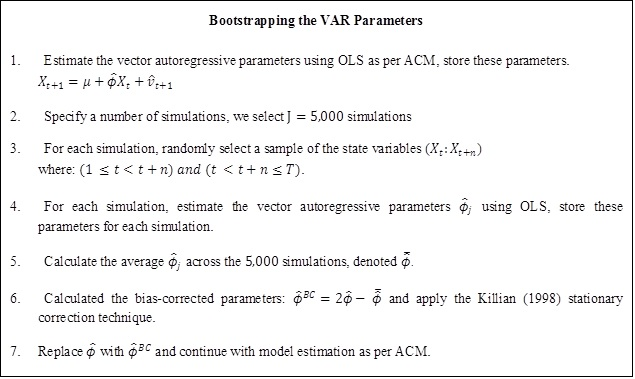## 4. Number of Factors

Multifactor DTSM’s typically use a number of factors that represent a reduced form expression of the yield curve; for ACM, factors are identified through principal component analysis. For many models, using principal component analysis or otherwise, typically at least three pricing factors are used to capture the level, slope and curvature dimensions of a yield curve (Kim Wright 2005). While the interpretation of high dimensional factors (greater than three) is not obvious, inclusion of additional factors may still be useful to the extent that there is an improvement to the fit of the model. For the ACM model fitted to US Treasury Bonds, five factors are employed to capture the multiple dimension of the yield curve. For a UK adaptation of ACM, Malik and Meldrum (2016) employ a four factor specification. For this application, we select five factors. As Table 1 indicates, the first five principal components (factors) can explain practically all of the cumulative proportion of yield curve variation. This means that five factors can be used to describe the yield curve with a very high degree of explanatory power. While more factors could be included that represent higher order (greater than five) principal components, the additional contribution to model results will be trivial and if included, could overfit the model.

Table 1: Principal Component Analysis

 Number of Factors Cumulative Explained Variation K=1 96.526% K=2 99.727% K=3 99.954% K=4 99.990% K=5 100.000% K=6 100.000% K=7 100.000%

The estimation implications for including a particular number of factors can be quantified by comparing observed yields at different maturities to fitted (model) yields at different maturities. Observed yields in this case refer to the zero-coupon yield data that is the input into the model. For example, comparing the daily five factor model’s 10 year fitted yield to the observed 10 year yield since the start of our time horizon (mid-1992), the fitting error is never greater than 5 basis points and is usually within 2.5 basis points (Figure 1).

Figure 1: Five Factor 10 Year Yield Fitting Error (observed minus fitted, basis points)To test the fitting errors across: (1) different maturity yields, (2) for estimation based on a varying number of factors and; (3) for different estimation techniques (OLS and bias-corrected), we calculate the root-mean-square error based on the daily observed yields and model fitted yields across the historical data set. Table 2 indicates that across all maturities, the marginal fitting improvement diminishes when moving from five factors to higher order factors; moreover, the OLS and bias-corrected model specifications have near identical fitting errors.

Table 2: Model RMSE

 OLS Model: Yield RMSE (basis points) Maturity (months) 2 factors 3 factors 4 factors 5 factors 6 factors 7 factors 12 12.314 9.229 7.656 1.057 0.059 0.010 24 18.268 5.570 4.247 0.745 0.031 0.007 60 28.006 4.070 1.147 0.945 0.046 0.008 120 25.472 4.898 3.328 0.925 0.085 0.011 Bias-Corrected Model: Yield RMSE (basis points) Maturity (months) 2 factors 3 factors 4 factors 5 factors 6 factors 7 factors 12 12.312 9.229 7.656 1.057 0.059 0.010 24 18.268 5.570 4.247 0.745 0.031 0.007 60 28.009 4.069 1.147 0.945 0.046 0.008 120 25.462 4.898 3.328 0.926 0.085 0.011

## 5. Model Results

Fitting the five factor ACM model to the Australian Treasury Bond term structure, it is apparent that a bootstrapped bias-corrected estimation technique gives materially different term premium and risk-neutral rate estimations relative to the OLS technique. Consistent with BRW, we find that bias-corrected term structure estimation will result in more variability in risk-neutral rates and consequently, more stable term premium estimations (Figures 2 and 3).

### Figure 2: 10 Year Term Premium Estimates (basis points)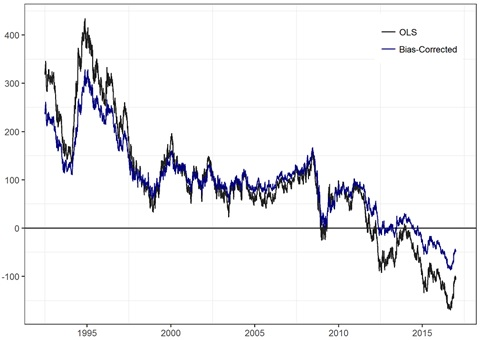### Figure 3: 10 Year Risk Neutral Estimates (percentage points)As the OLS and bias-corrected techniques result in different estimations of term premium and of risk-neutral yields, selecting a preferred estimation approach has important implications when determining the degree to which expectations or term premium have contributed to changes in Australian Treasury Bond yields over time. Over our sample period (mid-1992 to end-2016), there has been a secular decline in Treasury Bond yields within Australia which has also been experienced by a number of other developed countries over this time period (Bauer et al. 2013). As Figure 4 illustrates, the ACM OLS decomposition suggests that the most significant explanatory factor is a decline in term premium, with a smaller component attributable to lower risk-neutral rates. In comparison, the ACM bootstrapped decomposition, while similarly suggesting that a decline in the term premium is the most significant factor, attributes a larger contribution from declines in risk-neutral rates. This suggests that diminishing expectations for inflation or for real yields may account for a greater degree of the secular decline (Figure 5). Regardless of which estimation approach is preferred, both indicate that the term premium is the most significant contributor to the secular decline in yields experienced within Australia, but the degree varies.

### Figure 4: 10 Year Australian Treasury Bond Zero-Coupon Yield ACM OLS Decomposition (percentage points)### Figure 5: 10 Year Australian Treasury Bond Zero-Coupon Yield ACM Bootstrapped Bias-Correction Decomposition(percentage points)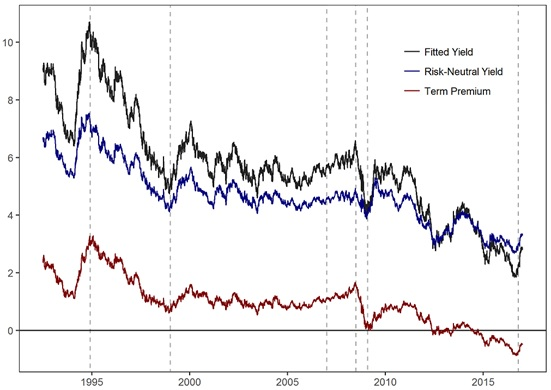For the period from 1995 to 1999, model results (figure 4 and 5) estimate a decline in term premium and a moderate decline in risk-neutral rates. This period coincided with the implementation of inflation targeting by the Reserve Bank of Australia. Implicit references to such an objective were made in 1989 and by mid-1993 it is considered that an inflation targeting objective was more explicit (Stevens 1999). A declining term premium estimate following on from an explicit inflation targeting objective is likely to be related to a reduction in the uncertainty of future inflation as central bank credibility is built over time. Moreover, an accompanying moderate decline in risk-neutral rates would likely reflect downwards revisions for future inflation as inflation is brought into a target band.

Following on from the late-1990’s, model results suggest term premium remained reasonably stable up until 2007 which may reflect the Reserve Bank retaining credibility in terms of achieving an inflation targeting mandate, that is by keeping inflation expectations steady. For the period prior to the Global Financial Crisis, model estimates suggests the term premium was gradually rising while risk-neutral rates remained constant. The economic intuition of this is not entirely clear given a Terms of Trade boom was occurring at the time. Throughout this period, increasing expectations for inflation, and consequently rising risk-neutral rates would be expected to contribute to yield movements, not necessarily the term premium.

During the Global Financial Crisis, model results suggest a sharp and sudden decline in term premium that could be attributed to a flight to quality, reflecting a shift out of risk assets and into safe haven assets such as Australian Treasury Bonds during a period of heightened risk.

The model results from 2010 to late 2016 suggest a period of further decline in the term premium. During this time, a number of major central banks undertook unconventional monetary policy initiatives such as large scale asset purchase programs. This has led to the total assets held by several major central banks (Federal Reserve, European Central Bank, Bank of Japan) growing from less than 4 trillion US dollars at the start of 2008 to more than 12 trillion US dollars as at November 2017 (Yardini Research). Such a large expansion of major central bank balance sheets presents a significant new source of demand for Government Debt Securities. Through this channel, the term premium is likely to fall for bonds purchased within these programmes and second order effects might include term premium (and other risk premia including equity risk premia) falling for other securities, such as Australian Treasury Bonds.

Model results now show negative term premium estimates that might appear puzzling if viewed from the prism of a risk averse investor requiring compensation. However, with consideration also given to other effects influencing the term premium, such as large scale asset purchases, a negative term premium is entirely plausible. While the term premium remains close to historic lows, a sharp increase in November 2016 coincided with a broad-based sell off in fixed income assets globally following the US election.

While both estimation approaches lead to broad consensus that a decline in term premium has been the major factor of a secular decline in Australian Treasury yields, the degree of variation attributed to risk-neutral rates and term premium estimates differ. As to the question of which approach is preferred, some research has suggested that bias-corrected parameters may improve DTSM point estimations (BRW, Diez de los Rios 2014). Other research suggests that applying bias-correction techniques might lead to less plausible term premium and risk-neutral yield point estimates compared to OLS estimation (Wright 2014, Malik and Meldrum 2016, Guimaraes 2014). While there is not necessarily consensus as to whether bias-correction techniques are preferred in improving DTSM parameter estimation, there is broad consensus that there is uncertainty in the estimation of DTSM parameters. Given this uncertainty, it is likely that the true data generating process is likely to be somewhere between the OLS estimated parameters and the bias-corrected parameters. As such, rather than suggesting an optimal estimation technique, we estimate a daily Australian Treasury Bond decomposition based on both techniques.

Given the uncertainty of estimation, the best point estimate might actually be a weighted combination of the OLS and bias-corrected estimation techniques. While in this paper we have not proposed a method that could be used to find the optimal combination of the OLS and bias-corrected approaches, development of such an optimisation process could be valuable in managing the uncertainty of coefficient estimations and thus allow for greater robustness in term premium and risk-neutral yield estimation. Alternatively, other approaches could be taken such as anchoring model-estimated expectations to survey data which provides additional information uncovering how risk-neutral yields evolve.

## 6. Conclusion

We have fitted a linear regression based DTSM to the Australian Treasury Bond term structure and estimated term premium through a daily yield decomposition. While our estimation approach follows ACM, we consider an alternative estimation approach that corrects for the potential bias inherent within a vector autoregressive system when variables are highly persistent. Our findings show that this alternative specification leads to significantly different term premium estimations and more variability in risk-neutral yields. Given this variation in estimation, we provide and analyse yield decompositions with and without this alternative estimation approach and suggest that the true data generating process most likely lies somewhere within the bounds of these model specifications. However, regardless of which estimation approach is preferred, both indicate that the term premium is the most significant contributor to the secular decline in yields experienced within Australia, but the degree varies. In this paper, no attempt is made to suggest an optimal estimation approach. However, finding a preferred combination of these alternative estimation techniques could be valuable in managing the uncertainty of coefficient estimations and ultimately allow for greater robustness in term premium estimation.

## References

Adrian, T., Crump, R.K. and Moench, E., 2013. Pricing the term structure with linear regressions. Journal of Financial Economics110(1), pp.110-138.

Bauer, M.D., Rudebusch, G.D. and Wu, J.C., 2012. Correcting estimation bias in dynamic term structure models. Journal of Business & Economic Statistics30(3), pp.454-467.

Bauer, M.D., Rudebusch, G.D. and Wu, J.C., 2014. Term premia and inflation uncertainty: Empirical evidence from an international panel dataset: Comment. The American Economic Review104(1), pp.323-337

Campbell, J.Y. and Shiller, R.J., 1991. Yield spreads and interest rate movements: A bird's eye view. The Review of Economic Studies58(3), pp.495-514.

Cox, J.C., Ingersoll Jr, J.E. and Ross, S.A., 1985. A theory of the term structure of interest rates. Econometrica: Journal of the Econometric Society, pp.385-407.

Dai, Q. and Singleton, K.J., 2002. Expectation puzzles, time-varying risk premia, and affine models of the term structure. Journal of financial Economics63(3), pp.415-441.

Duffie, D. and Kan, R., 1996. A yield‐factor model of interest rates. Mathematical finance6(4), pp.379-406.

Duffee, G.R., 2002. Term premia and interest rate forecasts in affine models. The Journal of Finance57(1), pp.405-443.

Fama, E.F. and MacBeth, J.D., 1973. Risk, return, and equilibrium: Empirical tests. The journal of political economy, pp.607-636.

Finlay, R. and Olivan, D., 2012. Extracting Information from Financial Market Instruments. The RBA Bulletin March Quarter, pp.45

Finlay, Richard, and Mark Chambers. "A term structure decomposition of the Australian yield curve." Economic Record 85.271 (2009): 383-400.

Finlay, Richard, and Sebastian Wende. "Estimating Inflation Expectations with a Limited Number of Inflation-Indexed Bonds." International Journal of Central Banking (2012).

Hamilton, J. and Wu, J., 2010. Identification and estimation of affine term structure models. Working paper, University of California, San Diego.

Kim, D.H. and Orphanides, A., 2005. Term structure estimation with survey data on interest rate forecasts.

Kim, D.H. and Orphanides, A., 2007. The bond market term premium: what is it, and how can we measure it?. BIS Quarterly Review, June.

Kim, D.H. and Wright, J.H., 2005. An arbitrage-free three-factor term structure model and the recent behavior of long-term yields and distant-horizon forward rates.

Kilian, L., 1998. Small-sample confidence intervals for impulse response functions. Review of economics and statistics, 80(2), pp.218-230.

Krippner, L. and Callaghan, M., 2016. Short-term risk premiums and policy rate expectations in the United States. Reserve Bank of New Zealand Analytical Note Series

Malik, S. and Meldrum, A., 2014. Evaluating the robustness of UK term structure decompositions using linear regression methods. Bank of England Working paper No.518

Malik, S. and Meldrum, A., 2016. Evaluating the robustness of UK term structure decompositions using linear regression methods. Journal of Banking & Finance67, pp.85-102.

Nelson, C.R. and Siegel, A.F., 1987. Parsimonious modeling of yield curves. Journal of business, pp.473-489.

Pope, A.L., 1990. Biases of estimators in multivariate non-Gaussian autoregression. Journal of Time Series Analysis11(3), pp.249-258.

Glenn, S. (1999). Six Years of Inflation Targeting. (Economic Society of Australia, Speech)

Guimarães, R., 2014. Expectations, risk premia and information spanning in dynamic term structure model estimation. Bank of England Working paper No.489

Svensson, L.E., 1994. Estimating and interpreting forward interest rates: Sweden 1992-1994 (No. w4871). National bureau of economic research.

Vasicek, O., 1977. An equilibrium characterization of the term structure. Journal of financial economics, 5(2), pp.177-188

Wright, J.H., 2011. Term premia and inflation uncertainty: Empirical evidence from an international panel dataset. The American Economic Review101(4), pp.1514-1534.

Wright, J.H., 2014. Term Premia and Inflation Uncertainty: Empirical Evidence from an International Panel Dataset: Reply. The American Economic Review104(1), pp.338-341.

Yardeni, E. (2017). Global Economic Briefing: Central Bank Balance Sheets. 1st ed. [ebook] Yardeni Research, Inc., p.1. Available at: http://www.yardeni.com/pub/peacockfedecbassets.pdf [Accessed 6 Jan. 2017].

## Footnotes

     The views expressed in this paper are those of the authors and do not necessarily reflect those of the Australian Office of Financial Management (AOFM) or the Australian Government.

     Portfolio Strategy and Research, Australian Office of Financial Management, Treasury Building, Langton Crescent, Parkes ACT 2600, Australia. The author is grateful for the interest, insights and comments provided by Michael Bath, Philip Drummond, Richard Finlay, Angelia Grant, Leo Krippner, Timothy McLennan-Smith and Matthew Wheadon. Thank you to Theodore Golat for assisting with initial work on this topic and to the authors of Pricing the term structure with linear regressions: Tobias Adrian, Richard Crump and Emmanuel Moench for providing useful code.

    While financial participant surveys can be considered direct measures of expectations, survey based measures are imperfect. Imperfections include the forecasts of participants not necessarily representing market-wide interest rate expectations captured within the prices of instruments such as government bonds. Moreover, surveys may be prone to a lack of incentive or the wrong incentive which may lead to a misrepresentation of views (Kim and Orphanides 2005). Another (imperfect) measure of expectations includes direct inference from instruments such as overnight indexed swaps. As with government bonds, these instruments include a term premium component (Krippner and Callaghan 2016).

    Hamilton and Wu (2010) detail the experience of those who have used these models and the significant numerical challenges that arise with the estimation of non-linear likelihood surfaces. In particular, the potential for parameter estimation not converging to the global maximum of the likelihood function is problematic.

    Risk-neutral in this context and throughout the paper refers to yields that would exist under the pure expectation hypothesis. That is yields with no price of risk (term premium).

    As BRW note, increasing the frequency of the data sample will not correct for a potential bias: while a higher frequency dataset does increase the number of observations it also increases the persistence.

    Anchoring model-estimated expectations to survey based measures is another approach that can be explored for managing DTSM VAR uncertainty. Survey data however is not without limitations including imperfections (Kim and Orphanides 2005) and availability. For example, for many countries outside of the US, interest rate surveys tend to be infrequent and are not always available out to long time horizons. However, Guimares (2014) does find robust estimation may still be possible with infrequent and imperfect survey data.

    This figure is based on OLS ACM estimation, not a bias-corrected estimation however both specifications give near identical fitting errors as indicated in Table 2.

    Estimations of decompositions can change as new data is included into the sample. This is because model parameter estimations based on regressions will change as the sample data changes.